American Journal of Applied Mathematics
Volume 3, Issue 6, December 2015, Pages: 305-311

Numerical Solution of a One Dimensional Heat Equation with Dirichlet Boundary Conditions

Benyam Mebrate

School of Mathematical and Statistical Sciences, Hawassa University, Hawassa, Ethiopia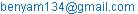Benyam Mebrate. Numerical Solution of a One Dimensional Heat Equation with Dirichlet Boundary Conditions. American Journal of Applied Mathematics. Vol. 3, No. 6, 2015, pp. 305-311. doi: 10.11648/j.ajam.20150306.20

Abstract: In this paper I present Numerical solutions of a one dimensional heat Equation together with initial condition and Dirichlet boundary conditions. Two methods are used to compute the numerical solutions, viz. Finite difference methods and Finite element methods. The finite element methods are implemented by Crank - Nicolson method. The numerical solutions of a one dimensional heat Equation together with initial condition and Dirichlet boundary conditions using finite difference methods do not always converge to the exact solutions. It indicates the occurrence of numerical instability in finite difference methods. Finally the numerical solutions obtained by these two methods are compared with the analytic solutions graphically into two and three dimensions.

Keywords: Initial Condition, Dirichlet Boundary Conditions, Finite Difference Methods, Finite Element Methods, Heat Equation, Instability

1. Introduction

Many physical problems in the real world such as heat equation, wave equation, Poisson equation and Laplace equation are modeled by partial differential equations. Some of these partial differential equations have exact solution in regular shape domain. But in general if the domain has irregular shape, computing exact solution of such equations is difficult. Due to this we use numerical methods to compute the solution of the modeled partial differential equations.

Finite difference and finite element methods are the two numerical methods that are applied to compute the solutions of partial differential equations by discretizing the domain in to finite number of regions. The solutions are computed at the grid points of the domain.

In literature  B-spline finite element methods are applied for numerical Simulation of one dimensional heat Equation. This motivates me to apply finite difference and finite element methods to heat equation with the given conditions and study the behavior of the solution for different values of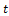.

This paper includes the following sections. Section 2 Problem definition Section 3 Finite difference methods Section 4 Finite element methods Section 5 Exact solution Section 6 Comparison of solutions Section 7 Conclusions.

2. Problem Definition

Partial differential equation of the form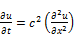(1)

is known as heat equation in one dimension. Equation (1) is parabolic second order linear partial differential equation.

The initial condition is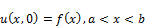(2)

and the Dirichlet boundary conditions are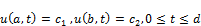(3)

Equations (1), (2) and (3) together are called Boundary – Initial value problem. To compute the numerical solution of equations (1) together with equations (2) and (3), we divide the interval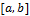in to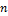equal parts by the points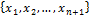with step length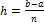. Also we divide the time interval in to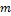equal parts by the points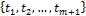with step length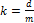. Here the problem is computing the solution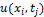for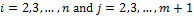. For simplicity we take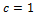.

3. Finite Difference Methods

In this method the partial differential equation is approximated by the set of finite difference equations. When we solve equation (1) using finite difference methods, infinitely many points in the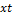- plane is replaced by a finite set of points. Thus equation (1) is replaced by algebraic equations and the algebraic equations are solved at each point. More specifically, we follow the following steps:

i.  We discretize the domain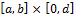.

ii.  We discretize equation (1). In this process equation (1) is replaced by algebraic equations.

iii.  We discretize the boundary conditions and the initial conditions.

iv. We solve the algebraic equations obtained in steps (ii) and (iii) at the points obtained in step (i).

Using these steps, we obtain the following set of algebraic equations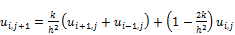(4)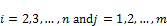Equation (4) approximates the partial differential equation (1) together with initial condition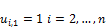(5)

and boundary conditions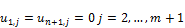(6)

The finite difference method for equation (1) together with equations (2) and (3) is stable if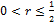and unstable if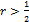.

4. Finite Element Methods

The main idea of finite element methods is changing equation (1) in to weak form. The solution of the weak formulation of equation (1) approximates the exact or analytic solution of equation (1). The accuracy of the solutions depends on the number and size of the elements, and the types of functions that are considered within the elements. To implement the finite element method for equation (1) we follow the following steps:

i.  We find the weak formulation of equation (1).

ii.  We discretize the interval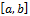and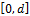.

iii.  We compute the stiffness matrix and mass matrix.

iv. We apply the Crank - Nicolson method to solve the resulting system of first order differential equations.

We use these steps as follows:

The weak formulation of equation (1) together with boundary conditions (3) is to find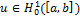such that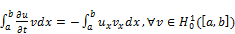(7)

Here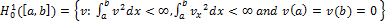Thus using (7) the finite element problem is to find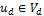such that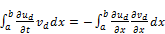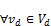(8)

where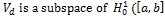and depends on a positive parameter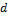. Also dim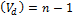.

Linear basis functions are chosen on the interval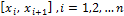as shown in the figure 1.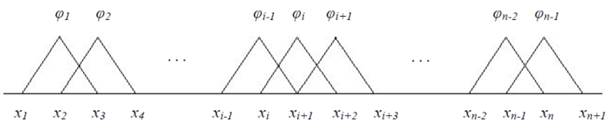Figure 1. Discretized domain and bases functions.

The basis function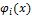is defined by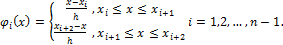Here we take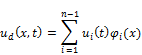and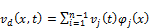(9)

Thus using (8) and (9) the weak formulation becomes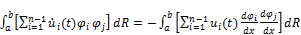(10)

We may write as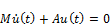(11)

Where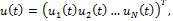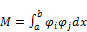(12)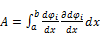(13)

Crank – Nicolson or trapezoidal method is applied to compute (11) at the nodes. The method is given by(14)

5. Numerical Solutions

In order to show the stability and instability of the finite difference method, and the accuracy of the numerical solution, we take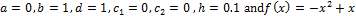. We also consider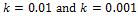. Then from equations (12) and (13),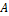and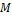are respectively.

Table 1. Stiffness matrix.

 20 -10 0 0 0 0 0 0 0 -10 20 -10 0 0 0 0 0 0 0 -10 20 -10 0 0 0 0 0 0 0 -10 20 -10 0 0 0 0 0 0 0 -10 20 -10 0 0 0 0 0 0 0 -10 20 -10 0 0 0 0 0 0 0 -10 20 -10 0 0 0 0 0 0 0 -10 20 -10 0 0 0 0 0 0 0 -10 20

and

Table 2. Mass matrix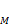.

 0.0667 0.0167 0 0 0 0 0 0 0 0.0167 0.0667 0.0167 0 0 0 0 0 0 0 0.0167 0.0667 0.0167 0 0 0 0 0 0 0 0.0167 0.0667 0.0167 0 0 0 0 0 0 0 0.0167 0.0667 0.0167 0 0 0 0 0 0 0 0.0167 0.0667 0.0167 0 0 0 0 0 0 0 0.0167 0.0667 0.0167 0 0 0 0 0 0 0 0.0167 0.0667 0.0167 0 0 0 0 0 0 0 0.0167 0.0667

Here we list the numerical solution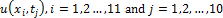of equation (1) together with initial condition (2) and boundary conditions (3).

5.1. Solutions Using Finite Difference Methods

Table 3. Solutions at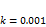.

 t x 0 0.1 0.2 0.3 0.4 0.5 0.6 0.7 0.8 0.9 1 0.000 0 0.0900 0.1600 0.2100 0.2400 0.2500 0.2400 0.2100 0.1600 0.0900 0 0.001 0 0.0880 0.1580 0.2080 0.2380 0.2480 0.2380 0.2080 0.1580 0.0880 0 0.002 0 0.0862 0.1560 0.2060 0.2360 0.2460 0.2360 0.2060 0.1560 0.0862 0 0.003 0 0.0846 0.1540 0.2040 0.2340 0.2440 0.2340 0.2040 0.1540 0.0846 0 0.004 0 0.0830 0.1521 0.2020 0.2320 0.2420 0.2320 0.2020 0.1521 0.0830 0 0.005 0 0.0816 0.1502 0.2000 0.2300 0.2400 0.2300 0.2000 0.1502 0.0816 0 0.006 0 0.0803 0.1483 0.1980 0.2280 0.2380 0.2280 0.1980 0.1483 0.0803 0 0.007 0 0.0791 0.1465 0.1960 0.2260 0.2360 0.2260 0.1960 0.1465 0.0791 0 0.008 0 0.0779 0.1447 0.1941 0.2240 0.2340 0.2240 0.1941 0.1447 0.0779 0 0.009 0 0.0768 0.1430 0.1921 0.2220 0.2320 0.2220 0.1921 0.1430 0.0768 0

Table 4. Solutions at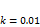.

 t x 0 0.1 0.2 0.3 0.4 0.5 0.6 0.7 0.8 0.9 1 0.00 0 0.0900 0.1600 0.2100 0.2400 0.2500 0.2400 0.2100 0.1600 0.0900 0 0.01 0 0.0700 0.1400 0.1900 0.2200 0.2300 0.2200 0.1900 0.1400 0.0700 0 0.02 0 0.0700 0.1200 0.1700 0.2000 0.2100 0.2000 0.1700 0.1200 0.0700 0 0.03 0 0.0500 0.1200 0.1500 0.1800 0.1900 0.1800 0.1500 0.1200 0.0500 0 0.04 0 0.0700 0.0800 0.1500 0.1600 0.1700 0.1600 0.1500 0.0800 0.0700 0 0.05 0 0.0100 0.1400 0.0900 0.1600 0.1500 0.1600 0.0900 0.1400 0.0100 0 0.06 0 0.1300 -0.0400 0.2100 0.0800 0.1700 0.0800 0.2100 -0.0400 0.1300 0 0.07 0 -0.1700 0.3800 -0.1700 0.3000 -0.0100 0.3000 -0.1700 0.3800 -0.1700 0 0.08 0 0.5500 0.7200 0.8500 -0.4800 0.6100 -0.4800 0.8500 -0.7200 0.5500 0 0.09 0 -1.2700 2.1200 -2.0500 1.9400 -1.5700 1.9400 -2.0500 2.1200 -1.2700 0

5.2. Solutions Using Finite Element Methods

Table 5. Solutions at.

 t x 0 0.1 0.2 0.3 0.4 0.5 0.6 0.7 0.8 0.9 1 0.000 0 0.0900 0.1600 0.2100 0.2400 0.2500 0.2400 0.2100 0.1600 0.0900 0 0.001 0 0.0877 0.1580 0.2080 0.2380 0.2480 0.2380 0.2080 0.1580 0.0877 0 0.002 0 0.0857 0.1560 0.2060 0.2360 0.2460 0.2360 0.2060 0.1560 0.0857 0 0.003 0 0.0840 0.1539 0.2040 0.2340 0.2440 0.2340 0.2040 0.1539 0.0840 0 0.004 0 0.0825 0.1519 0.2020 0.2320 0.2420 0.2320 0.2020 0.1519 0.0825 0 0.005 0 0.0811 0.1499 0.2000 0.2300 0.2400 0.2300 0.2000 0.1499 0.0811 0 0.006 0 0.0798 0.1480 0.1980 0.2280 0.2380 0.2280 0.1980 0.1480 0.0798 0 0.007 0 0.0786 0.1461 0.1959 0.2260 0.2360 0.2260 0.1959 0.1461 0.0786 0 0.008 0 0.0774 0.1443 0.1939 0.2240 0.2340 0.2240 0.1939 0.1443 0.0774 0 0.009 0 0.0763 0.1425 0.1920 0.2220 0.2320 0.2220 0.1920 0.1425 0.0763 0

Table 6. Solutions at.

 t x 0 0.1 0.2 0.3 0.4 0.5 0.6 0.7 0.8 0.9 1 0.00 0 0.0900 0.1600 0.2100 0.2400 0.2500 0.2400 0.2100 0.1600 0.0900 0 0.01 0 0.0742 0.1409 0.1902 0.2200 0.2300 0.2200 0.1902 0.1409 0.0742 0 0.02 0 0.0665 0.1252 0.1714 0.2004 0.2102 0.2004 0.1714 0.1252 0.0665 0 0.03 0 0.0594 0.1130 0.1549 0.1817 0.1910 0.1817 0.1549 0.1130 0.0594 0 0.04 0 0.0537 0.1019 0.1402 0.1647 0.1731 0.1647 0.1402 0.1019 0.0537 0 0.05 0 0.0485 0.0923 0.1268 0.1491 0.1568 0.1491 0.1268 0.0923 0.0485 0 0.06 0 0.0439 0.0834 0.1149 0.1350 0.1419 0.1350 0.1149 0.0834 0.0439 0 0.07 0 0.0397 0.0755 0.1039 0.1222 0.1285 0.1222 0.1039 0.0755 0.0397 0 0.08 0 0.0359 0.0684 0.0941 0.1106 0.1163 0.1106 0.0941 0.0684 0.0359 0 0.09 0 0.0325 0.0619 0.0852 0.1001 0.1053 0.1001 0.0852 0.0619 0.0325 0

6. Exact Solution

Using separation of variables the exact solution of equation (1) with initial condition (2) and boundary conditions (3) is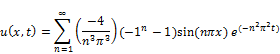The value of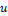at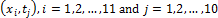is given in table 7 and 8 for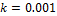and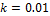respectively.

Table 7. Solutions at.

 t x 0 0.1 0.2 0.3 0.4 0.5 0.6 0.7 0.8 0.9 1 0.000 0 0.0900 0.1600 0.2100 0.2400 0.2500 0.2400 0.2100 0.1600 0.0900 0.0000 0.001 0 0.0880 0.1580 0.2080 0.2380 0.2480 0.2380 0.2080 0.1580 0.0880 0.0000 0.002 0 0.0861 0.1560 0.2060 0.2360 0.2460 0.2360 0.2060 0.1560 0.0861 0.0000 0.003 0 0.0845 0.1540 0.2040 0.2340 0.2440 0.2340 0.2040 0.1540 0.0845 0.0000 0.004 0 0.0829 0.1520 0.2020 0.2320 0.2420 0.2320 0.2020 0.1520 0.0829 0.0000 0.005 0 0.0815 0.1501 0.2000 0.2300 0.2400 0.2300 0.2000 0.1501 0.0815 0.0000 0.006 0 0.0802 0.1482 0.1980 0.2280 0.2380 0.2280 0.1980 0.1482 0.0802 0.0000 0.007 0 0.0789 0.1464 0.1960 0.2260 0.2360 0.2260 0.1960 0.1464 0.0789 0.0000 0.008 0 0.0778 0.1446 0.1941 0.2240 0.2340 0.2240 0.1941 0.1446 0.0778 0.0000 0.009 0 0.0767 0.1428 0.1921 0.2220 0.2320 0.2220 0.1921 0.1428 0.0767 0.0000

Table 8. Solutions at.

 t x 0 0.1 0.2 0.3 0.4 0.5 0.6 0.7 0.8 0.9 1 0.00 0 0.0900 0.1600 0.2100 0.2400 0.2500 0.2400 0.2100 0.1600 0.0900 0.0000 0.01 0 0.0756 0.1411 0.1902 0.2200 0.2300 0.2200 0.1902 0.1411 0.0756 0.0000 0.02 0 0.0668 0.1260 0.1718 0.2005 0.2102 0.2005 0.1718 0.1260 0.0668 0.0000 0.03 0 0.0598 0.1134 0.1554 0.1821 0.1912 0.1821 0.1554 0.1134 0.0598 0.0000 0.04 0 0.0539 0.1024 0.1407 0.1652 0.1736 0.1652 0.1407 0.1024 0.0539 0.0000 0.05 0 0.0488 0.0927 0.1275 0.1497 0.1574 0.1497 0.1275 0.0927 0.0488 0.0000 0.06 0 0.0441 0.0839 0.1155 0.1357 0.1427 0.1357 0.1155 0.0839 0.0441 0.0000 0.07 0 0.0400 0.0760 0.1046 0.1230 0.1293 0.1230 0.1046 0.0760 0.0400 0.0000 0.08 0 0.0362 0.0689 0.0948 0.1114 0.1171 0.1114 0.0948 0.0689 0.0362 0.0000 0.09 0 0.0328 0.0624 0.0859 0.1009 0.1061 0.1009 0.0859 0.0624 0.0328 0.0000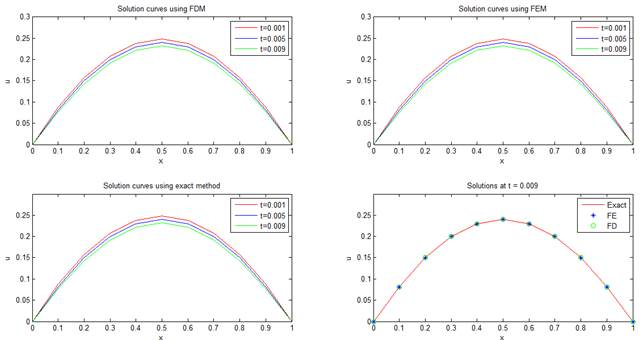Figure 2. Numerical solution curves with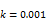.

7. Comparisons of Solutions

Here we compare the solutions using finite element methods, finite difference methods and exact method forand different values ofas shown in Figure 2 (a), (b) and (c). We also compare the numerical solution with the exact solution for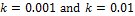as shown in Figure 2 (d) and Figure 3.

As we observe in Figure 2 (a), (b) and (c) the solution curve close to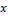– axis, that is,decreases asincreases. In figure 2 (d) the numerical solutions approximates the exact or analytic solutions. We can also see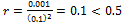.

As we see in Figure 3 the numerical solutions using finite element methods approximates the exact or analytic solutions but numerical solutions using finite difference method does not approximate the exact or analytic solutions. This is the effect of instability and not a truncation effect. Here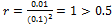.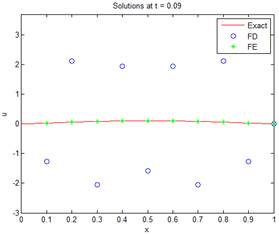Figure 3. Comparison of numerical solutions at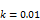.

In three dimensions the solution surface are shown in figure 4, 5 and 6.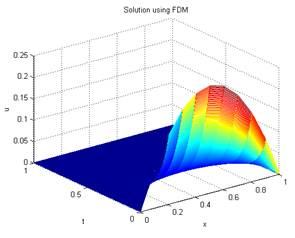(a)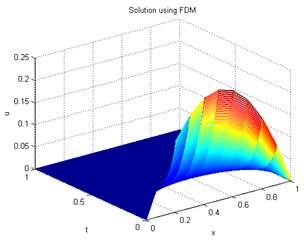(b)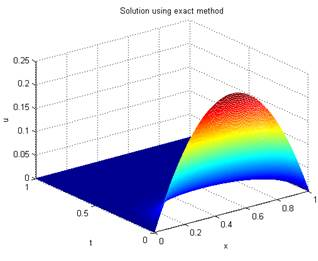(c)

Figure 4. Solution withand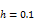.

As we see Figure (4) the graphs are identical. This indicates the numerical solutions approximate the exact solutions.

In figure 5 the surface (a) is drawn on the region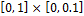because the solution using finite difference methods on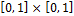is unbounded.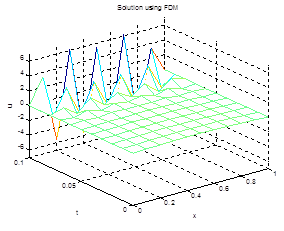(a)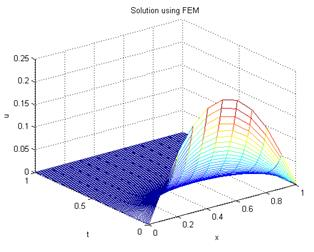(b)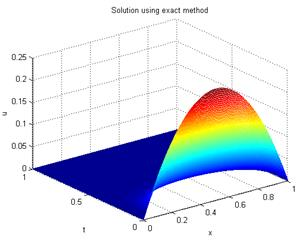(c)

Figure 5. Solutionwithand.

As we notice in figure 5, (a) is different from (b) and (c). This arises due to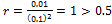. Hence the finite difference methods do not give approximate solutions to the exact solutions when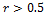.

8. Conclusions

The finite element methods approximate the exact solution of heat equation (1) together with (2) and (3). But the finite difference methodsdo not give approximate solutions for all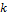.The stepsize in thedirection must be much smaller than the step size in thedirection.Though these depend on the partial differential equation as well as the boundary conditions, iffor the heat equation, then the finite difference method is stable, that is it gives reasonable approximations to the exact solution. If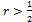, the finite difference method is unstable. Therefore; finite element methods are best methods if we compare with finite difference methods to compute the solutions of equation (1) together with (2) and (3).

References

1. Alfio Quarteroni, Numerical models for differential problems(2nd edition), Springer- Verlag, Italia, 2014.
2. Chapra Canal, Numerical methods for engineers (4th edition), The McGraw Hill Companies, 2001.
3. Erwin Kreyzing, Advanced Engineering Mathematics (9thedition), 2006 John Wiley and Sons, Inc.
4. G. Evans, J. Blackledge and P. Yardley,Numerical methods for partial differential equations, Springer-Verlag London Limited 2000.
5. Ioannis P Starroulakis and Stepan A Tersian, Partial Differential equations: An introduction with Mathematica and MAPLE(2nd edition), 2004 by world scientific publishing Co. Plc. Ltd.
6. J. David Logan,A first course in DEs,2006 Springer Science + Business Media. Inc.
7. Lichard L. Burden and J. Douglas Faires, Numerical analysis (9th edition), 2011, 2005, 2001 Brooks/Cole, Cengage Learning, 20 Channel Center StreetBoston, MA02210, USA.
8. Ravi P.Agarwal and Donal O'Regan, Ordinary and Partial DEs, 2006 Springer Science + Business Media, LLC(2009).
9. Susan C.Brenner, L. Ridgway Scott,The mathematical theory of finite element methods (3rdedition), 2008 Springer Sciences + Business Media, LLC.
10. V. Dabral, S. Kapoor and S. Dhawan, Numerical Simulation of one dimensional Heat Equation: B-Spline Finite Element Method,V.Dabral et al. / Indian Journal of Computer Science and Engineering (IJCSE), Vol. 2 No. 2 Apr-May 2011.

 Contents 1. 2. 3. 4. 5. 5.1. 5.2. 6. 7. 8.
Article ToolsAbstractPDF(328K)